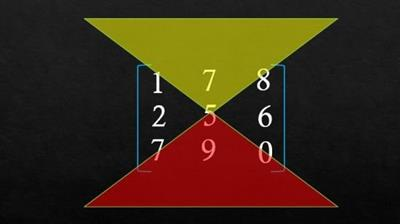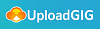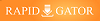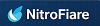Udemy - Complete Linear Algebra from Basics (NO REGRET)

Category: Tutorial

Posted on 2021-08-10, by voska89.

DescriptionMP4 | Video: h264, 1280x720 | Audio: AAC, 44.1 KHz, 2 Ch
Genre: eLearning | Language: English + srt | Duration: 80 lectures (7h 28m) | Size: 5.77 GB
Linear Algebra prerequisite for Machine Learning, Computer Science, Data Science, Big Data. Master matrix Algebra

What you'll learn:
Classification of matrices
Matrix algebra like addition, subtraction and multiplication
Trace of matrix,transpose of matrix
symmetric and skew symmetric matrix
Real and complex matrices
Orthogonal matrix
Determinant of Matrix: Minors, Cofactors of matrix
Invertible and non Invertible matrix
Inverse of matrix: adjoint of matrix
Finding the Rank of matrices
Row Echlon form
Relation between Rank and vectors of matrix
Linearly independant and dependant vectors
Vector space: Dimension, Basis, Span and Nullity
Solving simultaneous system of linear Equations
Homogeneous and non homogeneous system of equation
Eigen values and their carrosponding Eigen vectors
Properties of eigen values and eigen vector
cayley hamilton theorem
Requirements
A passion to learn about Matrices and Vectors
Fundamentals of Math, like arithmetic (addition, subtraction, multiplication, division) of positive and negative numbers, fractions, and decimals.
Ability to solve linear equations in two and three variable and graphing, and factoring, plus exponents and roots.
Description
Why study linear algebra?
Linear algebra is vital in multiple areas of science in general. Because linear equations are so easy to solve, practically every area of modern science contains models where equations are approximated by linear equations (using Taylor expansion arguments) and solving for the system helps the theory develop. Beginning to make a list wouldn't even be relevant ; you and I have no idea how people abuse of the power of linear algebra to approximate solutions to equations. Since in most cases, solving equations is a synonym of solving a practical problem, this can be VERY useful. Just for this reason, linear algebra has a reason to exist, and it is enough reason for any scientific to know linear algebra.
More specifically, in mathematics, linear algebra has, of course, its use in abstract algebra ; vector spaces arise in many different areas of algebra such as group theory, ring theory, module theory, representation theory, Galois theory, and much more. Understanding the tools of linear algebra gives one the ability to understand those theories better, and some theorems of linear algebra require also an understanding of those theories ; they are linked in many different intrinsic ways.
Outside of algebra, a big part of analysis, called functional analysis, is actually the infinite-dimensional version of linear algebra. In infinite dimension, most of the finite-dimension theorems break down in a very interesting way ; some of our intuition is preserved, but most of it breaks down. Of course, none of the algebraic intuition goes away, but most of the analytic part does ; closed balls are never compact, norms are not always equivalent, and the structure of the space changes a lot depending on the norm you use. Hence even for someone studying analysis, understanding linear algebra is vital.
In linear algebra, we will learn, Classification of matrices, Matrix algebra like addition,subtraction and multiplication, transpose of matrix, symmetric and skew symmetric matrix, Real and complex matrices, Determinant of Matrix: Minors, Cofactors of matrix, Inverse of matrix: adjoint of matrix, Finding the Rank of matrices, Row Echlon form, Relation between Rank and vectors of matrix, Linearly independant and dependant vectors, Vector space: Dimension, Basis, Span and Nullity, Solving system of linear Equations, Homogeneous and non homogeneous system of equation, Eigenvalues and their carrosponding Eigenvectors, Properties of eigenvalues and eigenvectors, cayley hamilton theorem.
Who this course is for
Students enrolled or planning to enroll in Linear Algebra class, and who want to excel in it
Anyone who needs Linear Algebra as a prerequisite for Machine Learning, Deep Learning, Artificial Intelligence, Computer Programming, Computer Graphics and Animation, Data Analysis, etc.
Engineers, Scientists and Mathematicians who want to work with Linear Systems and Vector Spaces
Professionals who need a refresher in Math, especially Algebra and Linear Algebra
Homepage
https://www.udemy.com/course/master-linear-algebra-for-machine-learning-data-science/Visit My Profile News Here : https://www.ebookee.com/user/voska89https://rapidgator.net/file/0a65a7682e9da3905fe8843a601e85bf/yuiy3.Complete.Linear.Algebra.from.Basics.NO.REGRET.part2.rar.html
https://rapidgator.net/file/a7f5c490bbefb5eb04d783b056896d40/yuiy3.Complete.Linear.Algebra.from.Basics.NO.REGRET.part3.rar.html
https://rapidgator.net/file/a1b41ff20c25a363c77923c1ddb21f57/yuiy3.Complete.Linear.Algebra.from.Basics.NO.REGRET.part4.rar.html
https://rapidgator.net/file/b54b4996303a206810c486f567601181/yuiy3.Complete.Linear.Algebra.from.Basics.NO.REGRET.part5.rar.html
https://rapidgator.net/file/fb0802b1dd9f6e1c26f255f679bf53a4/yuiy3.Complete.Linear.Algebra.from.Basics.NO.REGRET.part6.rar.html8331 dl's @ 2088 KB/s
7589 dl's @ 2933 KB/s
8193 dl's @ 2115 KB/s

Search More...
Udemy - Complete Linear Algebra from Basics (NO REGRET)Related Books